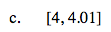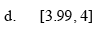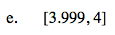### Home > PC > Chapter 9 > Lesson 9.1.3 > Problem9-37

9-37.
1. Now we want to calculate the average velocity of our toy car (with d(t) = 10t2). Find the average velocity on each of the intervals. Homework Help ✎

1. [4, 5]

2. [4, 4.1]

3. [4, 4.01]

4. [3.99, 4]

5. [3.999, 4]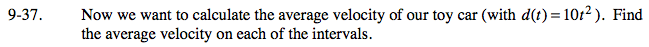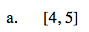$\frac{\Delta d}{\Delta t} = \frac{d(5) - d(4)}{5 - 4}$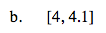$\frac{\Delta d}{\Delta t} = \frac{d(4.1) - d(4)}{4.1 - 4}$